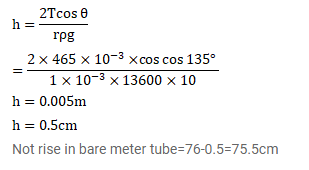Deepak Scored 45->99%ile with Bounce Back Crack Course. You can do it too!

# A barometer is constructed with its tube having radius

Question:

A barometer is constructed with its tube having radius $1.0 \mathrm{~mm}$. Assume that the surface of mercury in the tube is spherical in shape. If the atmospheric pressure is equal to $76 \mathrm{~cm}$ of mercury, what will be the height raised in the barometer tube. The contact angle of mercury with glass $=135^{\circ}$ and surface tension of mercury $=0.465 \mathrm{~N} / \mathrm{m}$. Density of mercury = $13600 \mathrm{~kg} / \mathrm{m}^{3}$.

Solution: